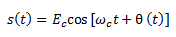# Introduction to Angle Modulation

## Introduction to Angle Modulation

In our previous articles, we have discussed the various types of Amplitude modulation systems in which the carrier amplitude is changed in accordance with the variation in the message signal magnitude .

There is another method of modulating a sinusoidal carrier namely the angle modulation in which either frequency or phase of the carrier is varied according to the message signal, but the carrier amplitude is constant .

Thus angle modulation system can be classified as follows :Frequency modulation as well as phase modulation are forms of angle modulation .

Angle modulation has several advantages over the amplitude modulation such as noise reduction, improved system fidelity and more efficient use of power.

But there are some disadvantages too such as increased bandwidth and use of more complex circuits.

Angle modulation is being used for the following applications :

2. TV sound transmission
5. Microwave communication
6. Satellite communication

The principle of angle modulation can be stated as follows :

The phase angle (Θ) of a sinusoidal carrier wave is varied with respect to time . An angle modulated wave can be expressed mathematically as :where Ec  is the peak carrier amplitude

ω=  carrier frequency

θ(t) = instantaneous phase deviation

In angle modulation θ(t) is a function of modulating signal .

This means that          θ(t) = F [em(t)]

Here, em(t) is the modulating signal given by ,

em = Esin (ωmt)